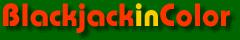# Chapter 2. Data by Count

We all know that the count is at the heart of card counting. Each card counting book tells us this in its own way. But do we fully feel the degree of impact on everything Blackjack?

"Beware the man of one book." — St. Thomas Aquinas

###How often do we get each True Count?

The basis of virtually all Blackjack card counting systems is the count. True counts require adjustment of the count by the undealt cards and are used by balanced strategies like Hi-Lo, Hi-Opt I, Omega II, Zen, Uston APC and Revere Point Count. Unbalanced strategies like KO, Red7 and KISS use the running count and avoid the additional calculation to find the true count. In this chapter, some charts will be provided for true counts and running counts — however not all charts will be provided for running counts.

Let's start by looking at the frequency of true counts. At the right we see the percentage of hands dealt at each count using Hi-Lo (one of the most common strategies). Note: There are several methods of calculating true counts as will be discussed later. Six-deck frequencies are displayed in red and two-deck frequencies in green. Obviously the most frequent count by far is zero. It is also clear that double-deck games experience substantially higher percentages of high and low counts. You will also see that the red line is much smoother than the green line. This is because these charts do not use the exact true counts. Players do not calculate the exact number of remaining cards when calculating TC. In this chart, remaining cards are estimated by half-decks. In double-deck games, this results in lower percentages of odd-numbered counts causing the jagged line that you see.

###Single-deck frequencies

Now let us look at single-deck. With only one deck the number of players becomes very important and can dramatically affect the results. Here the green line depicts frequencies for four players with two rounds. The red line displays two players with four rounds. You will note that with four players a true count of zero is far more likely. Also note that there are no rounds at TCs of 3, 7 and 9. Due to the method we use to calculate true counts, some counts cannot exist in single-deck. When you sit at a single-deck game, be aware that the number of players substantially affects the game.

###Cumulative frequencies

This chart provides the same data as the first chart, but cumulative. That is, each point provides the frequency that the count will be at least the number on the x-axis. We can see that for six decks (red line) we have a true count of zero or lower about 71% of the time. Depending on the rules, penetration, strategy, and decks, the count calls for an increased bet about 10%-35% of the time.

### Sim details

• Six decks, S17, DAS, LS, 4.81/6, Hi-Lo max indexes, trunc, half-deck
• Two decks, H17, DAS, 1.5/2, Hi-Lo Ill18 indexes, trunc, half-deck
• Single-deck, H17, 2 players, 4 rounds, Hi-Lo max indexes, trunc, quarter-deck
• Single-deck, H17, 4 players, 2 rounds, Hi-Lo max indexes, trunc, quarter-deck
• Ten billion rounds for six and two decks, five billion rounds for single-deck